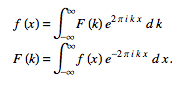# Fourier transform of a power signal or a voltage signal

## Homework Statement

By using fourier transform, I want to calculate power of signal. I confuse that f(x) in attached equation represents voltage or power. Is that possible when f(x) means power to use fourier transform.

## Homework Equations## Answers and Replies

RPinPA
Science Advisor
Homework Helper
In all of those equations, ##x(t)## is your original signal (not its power) and ##X(f)## or ##X_n## refer to the Fourier transform of ##x(t)##. Take the absolute value AFTER you Fourier Transform.